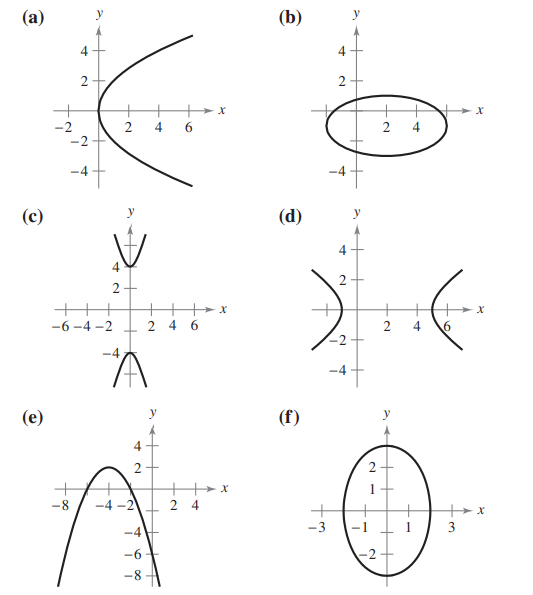×
Get Full Access to Calculus: Early Transcendental Functions - 6 Edition - Chapter 10.1 - Problem 5
Get Full Access to Calculus: Early Transcendental Functions - 6 Edition - Chapter 10.1 - Problem 5

×

# ?In Exercises 1–6, match the equation with its graph. [The graphs are labeled (a), (b), (c), (d), (e), and (f).] $$\ISBN: 9781285774770 141 ## Solution for problem 5 Chapter 10.1 Calculus: Early Transcendental Functions | 6th Edition • Textbook Solutions • 2901 Step-by-step solutions solved by professors and subject experts • Get 24/7 help from StudySoup virtual teaching assistantsCalculus: Early Transcendental Functions | 6th Edition 4 5 1 394 Reviews 11 0 Problem 5 In Exercises 1–6, match the equation with its graph. [The graphs are labeled (a), (b), (c), (d), (e), and (f).]\(\frac{x^{2}}{4}+\frac{y^{2}}{9}=1$$

Text Transcription:

x^2 / 4 + y^2 /  9 = 1

Step-by-Step Solution:

Step 1 of 5) Example 7 Apply l’Hôpital’s Rule to show that limxS0+ (1 + x)1>x = e. Solution The limit leads to the indeterminate form 1q. We let ƒ(x) = (1 + x)1>x and find limxS0+ ln ƒ(x). Since

Step 2 of 2

##### ISBN: 9781285774770

The full step-by-step solution to problem: 5 from chapter: 10.1 was answered by , our top Calculus solution expert on 11/14/17, 10:53PM. This full solution covers the following key subjects: . This expansive textbook survival guide covers 134 chapters, and 10738 solutions. Since the solution to 5 from 10.1 chapter was answered, more than 228 students have viewed the full step-by-step answer. Calculus: Early Transcendental Functions was written by and is associated to the ISBN: 9781285774770. This textbook survival guide was created for the textbook: Calculus: Early Transcendental Functions, edition: 6. The answer to “?In Exercises 1–6, match the equation with its graph. [The graphs are labeled (a), (b), (c), (d), (e), and (f).] $$\frac{x^{2}}{4}+\frac{y^{2}}{9}=1$$Text Transcription:x^2 / 4 + y^2 / 9 = 1” is broken down into a number of easy to follow steps, and 30 words.

## Discover and learn what students are asking

Chemistry: The Central Science : Molecular Geometry and Bonding Theories
?What is the distinction between a bond dipole and a molecular dipole moment?

Unlock Textbook Solution